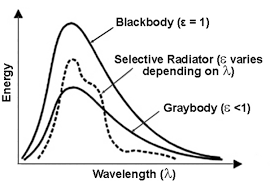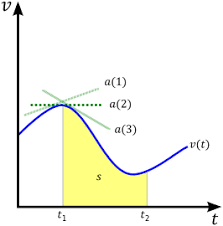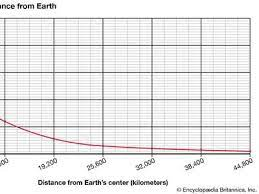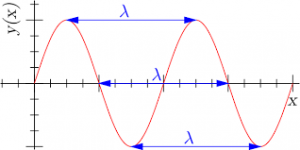## How to Calculate and Solve for Monochromatic Emissive Power | Radiation Heat TransferThe image above represents monochromatic emissive power.

To compute for monochromatic emissive power, five essential parameters are needed and these parameters are Planck’s Constant (h), Velocity of Light (c), Wavelength (λ), Boltzmann’s Constant (KB) and Temperature (T).

The formula for calculating monochromatic emissive power:

ebx = 2πhc²λ-5 / exp(ch/KBλT) – 1

Where:

ebx = Monochromatic Emissive Power | Planck’s Equation
h = Planck’s Constant
c = Velocity of Light
λ = Wavelength
KB = Boltzmann’s Constant
T = Temperature

Let’s solve an example;
Find the monochromatic emissive power when the planck’s constant is 6.626E-24, the velocity of light is 3E8, the wavelength is 22, the boltzmann’s constant is 1.380E-23 and the temperature is 10.

This implies that;

h = Planck’s Constant = 6.626E-24
c = Velocity of Light = 3E8
λ = Wavelength = 22
KB = Boltzmann’s Constant = 1.380E-23
T = Temperature = 10

ebx = 2πhc²λ-5 / exp(ch/KBλT) – 1
ebx = 2π(6.62607004e-34)(300000000)²(22)-5 / exp((300000000)(6.62607004e-34)/(1.38064852e-23)(22)(10)) – 1
ebx = 2π(6.62607004e-34)(90000000000000000)(1.94037e-7) / exp(1.987821012e-25/3.037426744e-21) – 1
ebx = 7.270512005456302e-23 / exp(0.00006544424539379114) – 1
ebx = 7.270512005456302e-23 / 1.0000654463869152 – 1
ebx = 7.270512005456302e-23 / 0.00006544638691519111
ebx = 1.11e-18

Therefore, the monochromatic emissive power is 1.11e-18.

## How to Calculate and Solve for Velocity | De Broglie’s LawThe image above represents velocity.

To compute for velocity, three essential parameters are needed and these parameters are Planck’s Constant (h), Wavelength (λ) and Mass (m).

The formula for calculating velocity:

v = h / λm

Where;

v = velocity
λ = wavelength
h = Planck’s constant
m = mass

Let’s solve an example;
Find the velocity when the wavelength is 10, the planck’s constant is 6.626e-34 and the mass is 5.

This implies that;

λ = wavelength = 10
h = Planck’s constant = 6.626e-34
m = mass = 5

v = h / λm
v = 6.62607004e-34 (10)(5)
v = 6.62607004e-34 (50)
v = 1.33e-35

Therefore, the velocity is 1.33e-35 m/s.

## How to Calculate and Solve for Mass | De Broglie’s LawThe image above represents mass.

To compute for mass, three essential parameters are needed and these parameters are Planck’s Constant (h), Wavelength (λ) and Velocity (v).

The formula for calculating the mass:

m = h / λv

Where;

m = mass
λ = wavelength
h = Planck’s constant
v = velocity

Let’s solve an example;
Find the mass when the wavelength is 4, the planck’s constant is 6.626e-34 and the velocity is 2.

This implies that;

λ = wavelength = 4
h = Planck’s constant = 6.626e-34
v = velocity = 2

m = hλv
m = 6.62607004e-34(4)(2)
m = 6.62607004e-34(8)
m = 8.28e-35

Therefore, the mass is 8.28e-35 kg.

## How to Calculate and Solve for Wavelength | De Broglie’s LawThe image above represents wavelength.

To compute for wavelength, three essential parameters are needed and these parameters are Planck’s Constant (h), Mass (m) and Velocity (v).

The formula for calculating wavelength:

λ = hmv

Where

λ = wavelength
h = Planck’s constant
m = mass
v = velocity

Let’s solve an example;
Find the wavelength when the planck’s constant is 6.62e-34, the mass is 21 and the velocity is 11.

This implies that;

h = Planck’s constant = 6.62e-34
m = mass = 21
v = velocity = 11

λ = hmv
λ = 6.62607004e-34(21)(11)
λ = 6.62607004e-34(231)
λ = 2.86e-36

Therefore, the wavelength is 2.86e-36 m.

Calculating the Mass when the Wavelength, the Planck’s Constant and the Velocity is Given.

m = h / λv

Where;

m = mass
λ = wavelength
h = Planck’s constant
v = velocity

Let’s solve an example;
Find the mass when the wavelength is 18, the planck’s constant is 6.626e-34 and the velocity is 6.

This implies that;

λ = wavelength = 18
h = Planck’s constant = 6.626e-34
v = velocity = 6

m = h / λv
m = 18 / 6.626e-34 x 6
m = 18 / 3.976e-33
m = 4.53

Therefore, the mass is 4.53 m.

## The Calculator Encyclopedia Calculates and Solves the Wavelength for a Wave – Particle Behaviour

According to Wikipedia,

Wave–particle duality is the concept in quantum mechanics that every particle or quantum entity may be partly described in terms not only of particles, but also of waves.

In physics, the wavelength is the spatial period of a periodic wave—the distance over which the wave’s shape repeats.

Nickzom Calculator requires two parameters to compute the wavelength of a wave or particle. These parameters are:

• Mass
• Velocity

The formula for computing the wavelength is:

λ = h / mv

Where:
λ = Wavelength
h = Planck’s Constant (6.63 x 10-34 js)
m = Mass
v = Velocity

Let’s solve an example, find the wavelength of a wave – particle with a mass of 300 Kg and a velocity of 3 x 1010 m/s.

From the example, we can see that:
m = 300
v = 3 x 1010

λ = 6.63 x 10-34 / 300 (3 x 1010)
λ = 6.63 x 10-34 / 9 x 1012
λ = 7.37 x 10-47

Therefore, the wavelength of the wave – particle (λ) is 7.37 x 10-47.